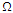# Electronic Devices - PNPN

### Exercise :: PNPN - General Questions

6.

How many terminals does a programmable unijunction transistor (PUT) have?

 A. 4 B. 3 C. 2 D. 1

Explanation:

No answer description available for this question. Let us discuss.

7.

What is the typical value of the reverse resistance of SCRs?

 A. 1to 10B. 100to 1 kC. 1 kto 50 kD. 100 kor more

Explanation:

No answer description available for this question. Let us discuss.

8.

The ISO-LIT Q1 16-pin Litronix opto-isolator DIP contains ________ opto-isolators.

 A. 4 B. 8 C. 12 D. 6

Explanation:

No answer description available for this question. Let us discuss.

9.

How many terminals does a Shockley diode have?

 A. 5 B. 4 C. 3 D. 2

Explanation:

No answer description available for this question. Let us discuss.

10.

What is the range of the variable resistor in the equivalent circuit of a unijunction transistor?

 A. 50to 5 kB. 6 kto 10 kC. 5to 50D. 1to 5